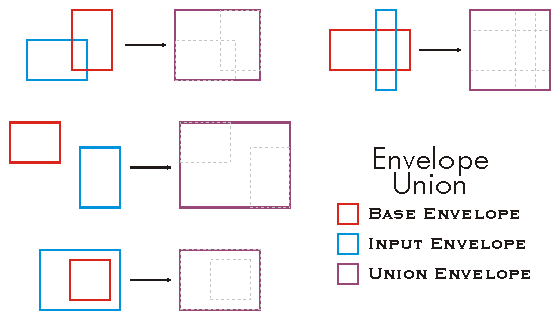ArcObjects Library Reference (Geometry)

# IEnvelope.Union Method

Adjusts to overlap inEnvelope.

```[Visual Basic .NET]
Public Sub Union ( _
ByVal inEnvelope As IEnvelope _
)```
```[C#]
public void Union (
IEnvelope inEnvelope
);```
```[C++]
HRESULT Union(
IEnvelope* inEnvelope
);```
```[C++]
Parameters
inEnvelope
inEnvelope is a parameter of type IEnvelope
```

#### Product Availability

Available with ArcGIS Engine, ArcGIS Desktop, and ArcGIS Server.

#### Description

Sets the Envelope equal to the union of the base Envelope and the input Envelope.  The XMin and YMin of the resulting Envelope is the minimum XMin and YMin respectively between the base and input Envelopes, and the XMax and YMax of the resulting Envelope is the maximum XMax and YMax respectively between the base and input Envelopes.

#### Remarks[C#]

public void Union()
{
IEnvelope envelope1 = new EnvelopeClass();
IEnvelope envelope2 = new EnvelopeClass();
envelope1.PutCoords(100, 100, 200, 200);
envelope2.PutCoords(150, 150, 250, 250);
envelope1.Union(envelope2);
IPoint lowerLeft = envelope1.LowerLeft;
IPoint lowerRight = envelope1.LowerRight;
IPoint upperLeft = envelope1.UpperLeft;
IPoint upperRight = envelope1.UpperRight;
String report = "LowerLeft  X = " + lowerLeft.X + "\n" +
"LowerLeft  Y = " + lowerLeft.Y + "\n\n" +
"LowerRight X =  " + lowerRight.X + "\n" +
"LowerRight Y =  " + lowerRight.Y + "\n\n" +
"UpperLeft  X = " + upperLeft.X + "\n" +
"UpperLeft  Y = " + upperLeft.Y + "\n\n" +
"UpperRight X =  " + upperRight.X + "\n" +
"UpperRight Y =  " + upperRight.Y;

System.Windows.Forms.MessageBox.Show(report);
}

[Visual Basic .NET]

' The example shows a union of 2 envelopes. The result is put in the first
' envelope and it will have the extent of both envelopes (100,100,250,250).

Public Sub t_EnvUnion()
Dim pEnv1 As IEnvelope
Dim pEnv2 As IEnvelope
pEnv1 = New Envelope
pEnv2 = New Envelope
pEnv1.PutCoords(100, 100, 200, 200)
pEnv2.PutCoords(150, 150, 250, 250)

pEnv1.Union(pEnv2)

Dim dXmin As Double, dYmin As Double, dXmax As Double, dYmax As Double
pEnv1.QueryCoords(dXmin, dYmin, dXmax, dYmax)

End Sub

#### .NET Snippets

Zoom to Selected Globe Features

#### .NET Samples

Curve conversion add-in (Code Files: CurveConversionDockWin) | Move a graphic along a path in ArcMap (Code Files: MapGraphicKeyframe) | RSS weather layer (Code Files: RSSWeatherLayerClass)

#### .NET Related Topics

How to find the combined extent of two geometries